Next: PHASE OF A MINIMUM-PHASE Up: PHASE DELAY AND GROUP Previous: Observation of dispersive waves

## Group delay of all-pass filters

We have already discussed (page) all-pass filters, i.e., filters with constant unit spectra. They can be written as.In the frequency domain, P(Z) can be expressed as, where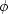is real and is called the phase shift." Clearly,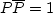for all real.It is an easy matter to make a filter with any desired phase shift--we merely Fourier transforminto the time domain. Ifis arbitrary, the resulting time function is likely to be two-sided. Since we are interested in physical processes that are causal, we may wonder what class of functionscorresponds to one-sided time functions. The answer is that the group delay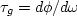of a causal all-pass filter must be positive.

Proof thatfor a causal all-pass filter is found in FGDP; there is no need to reproduce the algebra here. The proof begins from equation () and uses the imaginary part of the logarithm to get phase. Differentiation with respect toyields a form that is recognizable as a spectrum and hence is always positive.

A single-pole, single-zero all-pass filter passes all frequency components with constant gain and a phase shift that can be adjusted by the placement of the pole. Taking Z0 near the unit circle causes most of the phase shift to be concentrated near the frequency where the pole is located. Taking the pole farther away causes the delay to be spread over more frequencies. Complicated phase shifts or group delays can be built up by cascading single-pole filters.

The above reasoning for a single-pole, single-zero all-pass filter also applies to many roots, because the phase of each will add, and the sum of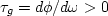will be greater than zero.

The Fourier dual to the positive group delay of a causal all-pass filter is that the instantaneous frequency of a certain class of analytic signals must be positive. This class of analytic signals is made up of all those with a constant envelope function, as might be approximated by field data after the process of automatic gain control.

## EXERCISES:

1. Let xt be some real signal. Let yt =xt+3 be another real signal. Sketch the phase as a function of frequency of the cross-spectrum X(1/Z)Y(Z) as would a computer that put all arctangents in the principal quadrants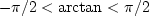.Label the axis scales.
2. Sketch the amplitude, phase, and group delay of the all-pass filter,whereandis small. Label important parameters on the curve.
3. Show that the coefficients of an all-pass, phase-shifting filter made by cascading(Z0 - Z) withare real.
4. A continuous signal is the impulse response of a continuous-time, all-pass filter. Describe the function in both time and frequency domains. Interchange the words time" and frequency" in your description of the function. What is a physical example of such a function? What happens to the statement, the group delay of an all-pass filter is positive?
5. A graph of the group delay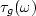shows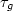to be positive for all.What is the area underin the range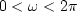? (HINT: This is a trick question you can solve in your head.)Next: PHASE OF A MINIMUM-PHASE Up: PHASE DELAY AND GROUP Previous: Observation of dispersive waves
Stanford Exploration Project
10/21/1998